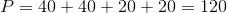# ISEE Lower Level Math : How to find the perimeter of a rectangle

## Example Questions

### Example Question #81 : Plane Geometry

If the perimeter of a rectangle is equal to 54 inches, what are possible values for the width and length?

Width of 10 inches and length of 16 inches

Width of 11 inches and length of 17 inches

Width of 10 inches and length of 17 inches

Width of 10 inches and length of 18 inches

None of these

Width of 10 inches and length of 17 inches

Explanation:

The perimeter of a rectangle is equal to: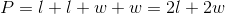The only answer choice that provides dimensions for which the perimeter of a rectangle would be 54 inches is when there is a width of 10 inches a length of 17 inches.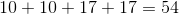Therefore, a width of 10 inches and length of 17 inches is the correct answer.

### Example Question #82 : Plane Geometry

If the width of a rectangle is 2 inches and the length is 6 inches, what is the perimeter in inches?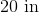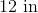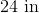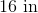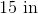Explanation:

The perimeter of a rectangle can be found by adding together all the sides. The formula is:Use the given values for the length and the width to solve.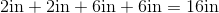16 inches is the correct answer.

### Example Question #83 : Plane Geometry

A rectangle has a width of 5 inches and an area of 35 inches. What is the length, in inches?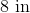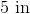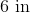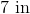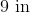Explanation:

The area of a rectangle is equal to the length times the width.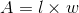Here, we know that the area is 35, and the width is 5. We can fill in part of the equation with these values.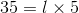The only number that equals 35 when multiplied by 5 is 7.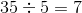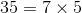### Example Question #84 : Plane Geometry

If the width of a rectangle is 3 inches and the length is 5 inches, what is the perimeter?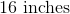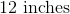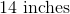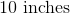Explanation:

The perimeter of a rectangle can be found by adding together all the sides. This would give us: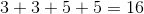Therefore, 16 inches is the correct answer.

### Example Question #11 : How To Find The Perimeter Of A Rectangle

Marcell wants to put a new fence around the perimeter of his garden. Marcell's rectangular garden is 30 feet long and 15 feet wide. How many feet of fence does Marcell need?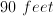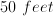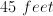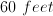Explanation: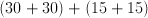### Example Question #12 : How To Find The Perimeter Of A Rectangle

The length of a rectangle is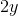, and the width of the rectangle is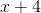. What is the perimeter if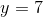and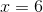?Explanation:

If the length of a rectangle isand the width is, the perimeter is: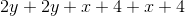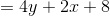Now plug inand: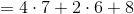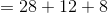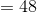### Example Question #13 : How To Find The Perimeter Of A Rectangle

If the perimeter of a rectangle isinches and its length is twice the value of its width, what is the value of the width of the rectangle in inches?inchesinchesinchesinchesinches

Explanation:

If the length of the rectangle is twice the value of its width, then if the width is equal to w, then the length is equal to 2w.

Given that the perimeter of a rectangle is equal to the value of all the sides added together, the following equation would apply: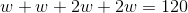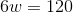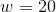This means that the rectangle isinches wide.

### Example Question #14 : How To Find The Perimeter Of A Rectangle

The area of a rectangle is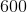. One of its sides is. What is its perimeter?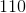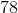Explanation:

Remember that the area of a rectangle is equal to its base times its height. If its area isand one side has a length of, we can find the other side by dividing these: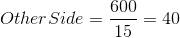So, our rectangle looks like this:The perimeter is easily calculated: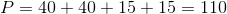### Example Question #15 : How To Find The Perimeter Of A Rectangle

What is the perimeter of the following rectangle?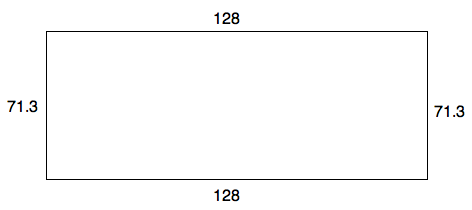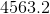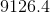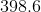631.1

199.3Explanation:

The perimeter of a rectangle is very easy to solve.  Merely add up all the sides: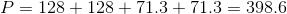### Example Question #16 : How To Find The Perimeter Of A Rectangle

One of a rectangle's sides has a length of. Another of its sides is half that length. What is its perimeter?Explanation:

If one side of the rectangle isand the other half of that, then the other side must be: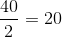Our rectangle therefore looks like this: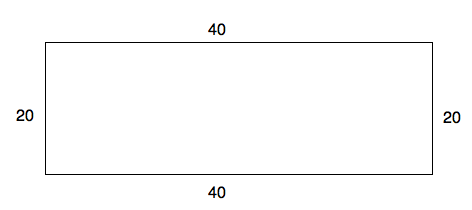The perimeter is calculated very easily.  Just sum up all the sides!